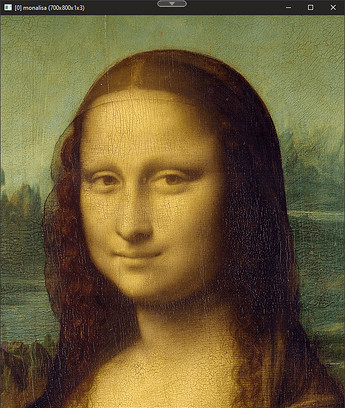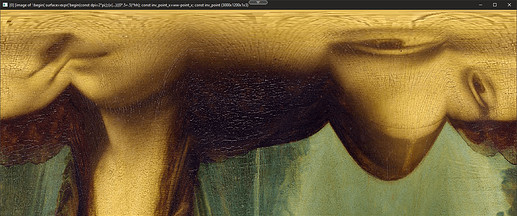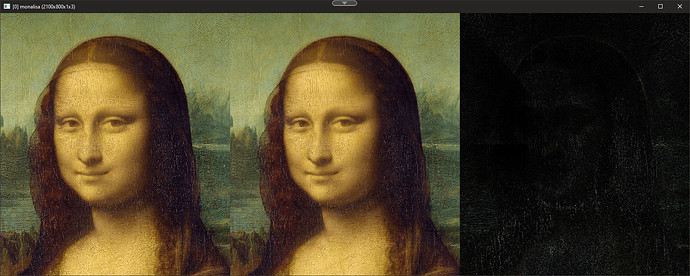Lossless rectangular-polar transformation

Right now, I am in a search of a lossless rectangular-polar transformation. To clarify, this is what rectangular-polar coordinate map looks like:So, these are the two axis of a rectangular-polar map. The x-axis ranges from 0-2*pi while the y-axis ranges from 0,1. It's like polar coordinates, but as a rectangle. However, in my case, I need to be able to shift the vertice at the center, and transform it forward and backward in a lossless matter.

My current solution using a interpretative language:

new_rep_recpoltrans:
skip $${1=0},$${2=0},$${3=0},$${4=}
if $$1<-1||$$1>1 error "($$1>=-1&&$$1<=1)=0" fi
if $$2<-1||$$2>1 error "($$2>=-1&&$$2<=1)=0" fi

#1 = X-Location of co-aligned vertices. The range is -1 to 1 where 0 is center of image. # #2 = Y-Location of co-aligned vertices. The range is -1 to 1 where 0 is center of image.#
#$$3 = 0=From rectangular-polar to cartersian standard | 1=To rectangular-polar# #$$4 = Defines the orientation the before image was. This can only be used if $3==0# repeat $$! l[$$>] ov=$${-rep_2dcr} if$$3 maxlength={max(w,h)*1.5} perimeter={(w+h)*2} {$$perimeter},{maxlength},{d},{s},":begin( surface=expr('begin(const dpi=2*pi;);(x/w)*dpi;',w); #x-coordinates in rectangular-polar are defined as in 0-2*pi range# const dpi=pi*2; const ww=w#0-1; #Pixel coordinates limits are defined by 0-(width of first image minus 1)# const hh=h#0-1; #Pixel coordinates limits are defined by 0-(width of first image minus 1)# const ihh=h-1; #This is used to convert from cartesian to rectangular-polar y-axis to 0-1 range# const point_x=round((($$1*-1)*.5+.5)*ww); #This takes into account of the fact that we're using pixel coordinates. Rounding is not needed in vector. Also used to find distance away.# const point_y=round(($2*.5+.5)*hh); #This takes into account of the fact that we're using pixel coordinates. Rounding is not needed in vector Also used to find distance away.#
const inv_point_x=ww-point_x; #Find the distance away from the center#
const inv_point_y=hh-point_y; #Find the distance away from the center#
const cut_ang_s0=abs(atan2(inv_point_y,inv_point_x)); #Draw 4 triangles where the center contains co-aligned vertices. This defines the top triangle#
const cut_ang_s1=pi-abs(atan2(inv_point_y,point_x)); #Draw 4 triangles where the center contains co-aligned vertices. This defines the right triangle#
const cut_ang_s2=pi+abs(atan2(point_y,point_x)); #Draw 4 triangles where the center contains co-aligned vertices. This defines the bottom triangle#
const cut_ang_s3=dpi-abs(atan2(point_y,inv_point_x)); #Draw 4 triangles where the center contains co-aligned vertices. This defines the left triangle#
distanceaway=[ww-point_x,hh-point_y,point_x,point_y]; #Vector of distance away#
);
surface_angle=surface[x];
surface_angle>cut_ang_s0&&surface_angle<=cut_ang_s1?side=1:
surface_angle>cut_ang_s1&&surface_angle<=cut_ang_s2?side=2:
surface_angle>cut_ang_s2&&surface_angle<=cut_ang_s3?side=3:
side=0;
mdist=abs(side%2?1/sin(surface_angle):1/cos(surface_angle));
dix=(point_x+cos(surface_angle)*distanceaway[side]*mdist*y/ihh);
diy=(point_y+sin(surface_angle)*distanceaway[side]*mdist*y/ihh);
I(#0,round(ww-dix),round(diy),z);"

k.
else

#The block below defines the dimension given orientation info#

#Block Begin#
$$=val orientation=$${val{$$>+4}} perimeter={w} length_1={perimeter-h*(4/3)} length_2={perimeter-length_1} if$$orientation
width={min($$length_1,$$length_2)}
height={max($$length_1,$$length_2)}
else
width={max($$length_1,$$length_2)}
height={min($$length_1,$$length_2)}
fi

#Block End#

v + echo {w},{h} v -
{$$width},{height},{d},{s},":begin( const ww=w#0-1; #Max pixel x-coordinate of rectangular-polar image# const hh=h#0-1; #Max pixel y-coordinate of rectangular-polar image# const nw=w-1; #Max pixel x-coordinate of new image# const nh=h-1; #Max pixel y-coordinate of new image# const sd=max(nw,nh)/min(nw,nh); #Max dimension. Unused for now# const dpi=2*pi; #Double pi as a constant# const cx=.5+$$1*.5; #Coordinate of coaligned vertice#
const cy=.5+2*.5; #Coordinate of coaligned vertice# const px=round(cx*nw); const py=round(cy*nh); const rpx=nw-px; const rpy=nh-py; ); xpos=x-px; ypos=y-py; ax=1-(atan2(ypos,xpos)+pi)/dpi; ay_x=x>px?(rpx-(nw-x))/rpx:(px-x)/px; ay_y=y>py?(rpy-(nh-y))/rpy:(py-y)/py; ay=max(ay_x,ay_y); I(#0,ax*ww,ay*hh,z); " r2dx. 50%,6 k. else error "3|"$"3!=intnum[-3,2]" fi endl done What does the mona lisa image looks like when you convert Cartesian coordinate to rectangular-polar coordinates? Original:$ sp monalisaAfter converting coordinates:

$sp monalisa rep_recpoltrans 0,0,1Test result of rectangular-polar transformation:$ sp monalisa +new_rep_recpoltrans 0,0,1 new_rep_recpoltrans. 0,0,0,1 +blend difference a xIf you look closely on the dark image, it’s clear that there’s information loss between the transformation. This thread should be about solving the information loss while still being useful for applying filters such as axis streak to do perspective streak.

There is a max difference of ~171.5. That is not good at all.

I have decided I consider this solved though it doesn't really answer the question.

Using this code:

new_rep_recpoltrans:
skip $${1=0},$${2=0},$${3=0},$${4=}
if $$1<-1||$$1>1 error "($$1>=-1&&$$1<=1)=0" fi
if $$2<-1||$$2>1 error "($$2>=-1&&$$2<=1)=0" fi

repeat $$! l[$$>]
if $$3 maxlength={max(w,h)*2} perimeter={(w+h)*4} {perimeter},{maxlength},{d},{s},":begin( surface=expr('begin(const dpi=2*pi;);(x/w)*dpi;',w); const dpi=pi*2; const ww=w#0-1; const hh=h#0-1; const ihh=h-1; const point_x=(($$1*-1)*.5+.5)*ww;
const point_y=($2*.5+.5)*hh; const inv_point_x=ww-point_x; const inv_point_y=hh-point_y; const cut_ang_s0=abs(atan2(inv_point_y,inv_point_x)); const cut_ang_s1=pi-abs(atan2(inv_point_y,point_x)); const cut_ang_s2=pi+abs(atan2(point_y,point_x)); const cut_ang_s3=dpi-abs(atan2(point_y,inv_point_x)); distanceaway=[ww-point_x,hh-point_y,point_x,point_y]; ); surface_angle=surface[x]; surface_angle>cut_ang_s0&&surface_angle<=cut_ang_s1?side=1: surface_angle>cut_ang_s1&&surface_angle<=cut_ang_s2?side=2: surface_angle>cut_ang_s2&&surface_angle<=cut_ang_s3?side=3: side=0; mdist=abs(side%2?1/sin(surface_angle):1/cos(surface_angle)); dix=(point_x+cos(surface_angle)*distanceaway[side]*mdist*y/ihh); diy=(point_y+sin(surface_angle)*distanceaway[side]*mdist*y/ihh); I(#0,round(ww-dix),round(diy),z);" k. else $$=val orientation=$${val{$$>+4}} perimeter={w} length_1={perimeter-h*2} length_2={perimeter-length_1} if$$orientation width={min($$length_1,$$length_2)} height={max($$length_1,$$length_2)} else width={max($$length_1,$$length_2)} height={min($$length_1,$$length_2)} fi {$$width/4},{height/4},{d},{s},":begin( const ww=w#0-1; const hh=h#0-1; const nw=w-1; const nh=h-1; const sd=max(nw,nh)/min(nw,nh); const dpi=2*pi; const cx=.5+$$1*.5; const cy=.5+$2*.5;
const px=cx*nw;
const py=cy*nh;
const rpx=nw-px;
const rpy=nh-py;
);
xpos=x-px;
ypos=y-py;
ax=1-(atan2(ypos,xpos)+pi)/dpi;
ay_x=x>px?(rpx-(nw-x))/rpx:(px-x)/px;
ay_y=y>py?(rpy-(nh-y))/rpy:(py-y)/py;
ay=max(ay_x,ay_y);
I(#0,ax*ww+(ax*ay),ay*hh,z,2);
"
k.
fi
endl done

I was able to achieve much better result than the one I have implemented in github/gmic-community/reptorian.gmic.